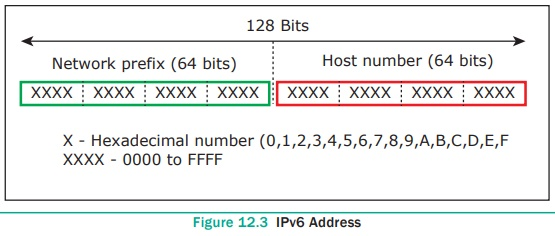## Chapter: 12th Computer Applications : Chapter 12 : DNS (Domain Name System)

Internet Protocol (IP) address is simply the logical address in the network layer.

Internet Protocol (IP) address is simply the logical address in the network layer. Like how the door number/flat number is used to differentiate individual house from others in the same apartment, IP address is also used to find the host system in the whole network. Due to increase in the number of system in a network there is a need of more addresses which lead to two addressing methods i.e., IPv4 and IPv6.

IPv4 address is a 32-bit unique address given to a computer system. No two systems can have same IP address. If the network has p connections then ‘ p’ addresses should be there. An address space is the total number of addresses that can be made by that protocol. It is determined by the number of bits that the protocol use. If the protocol uses ‘n’ bits then the address space of that protocol would be ‘2n’ addresses. So, the number of addresses that can be formed in IPv4 is 232. There are two ways to represent the IP address

● Binary notation

● Dotted-decimal notation

In binary notation the address is expressed as 32-bit binary values.

For E.g. 00111001 10001001 00111000 00000111

In dotted -decimal notation the address is written in decimal format separated by dots(.). Refer Figure 12.2

For e.g. 128.143.137.144